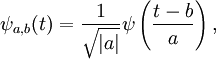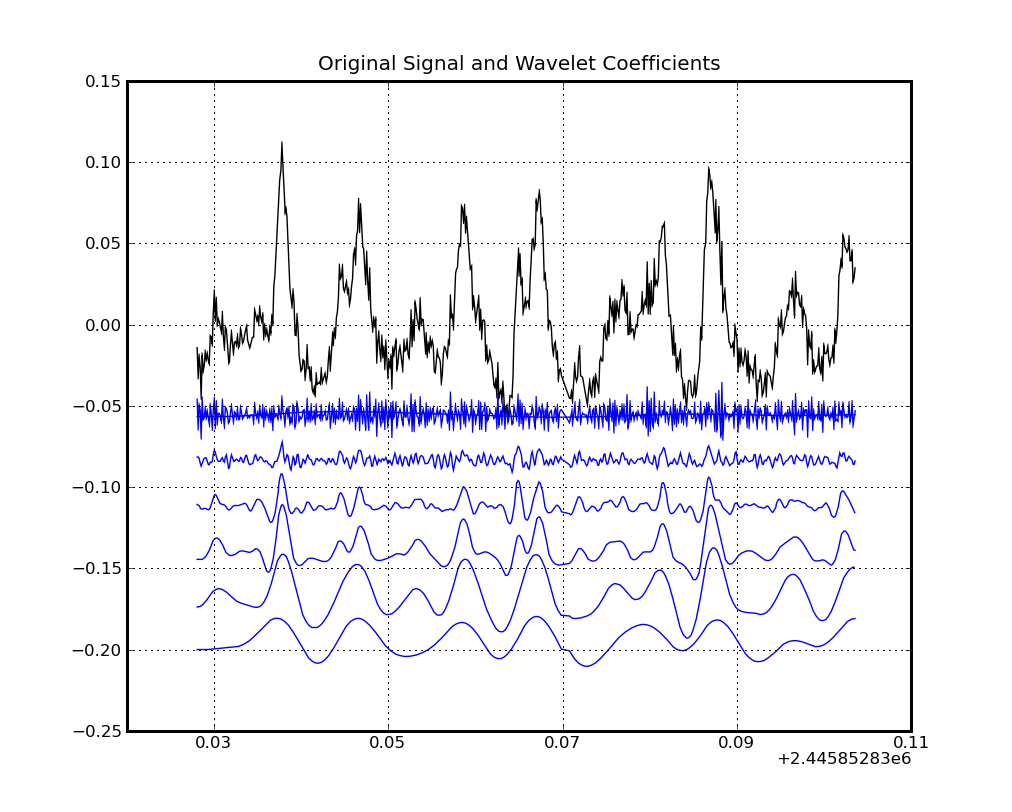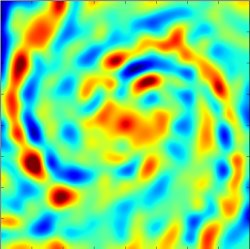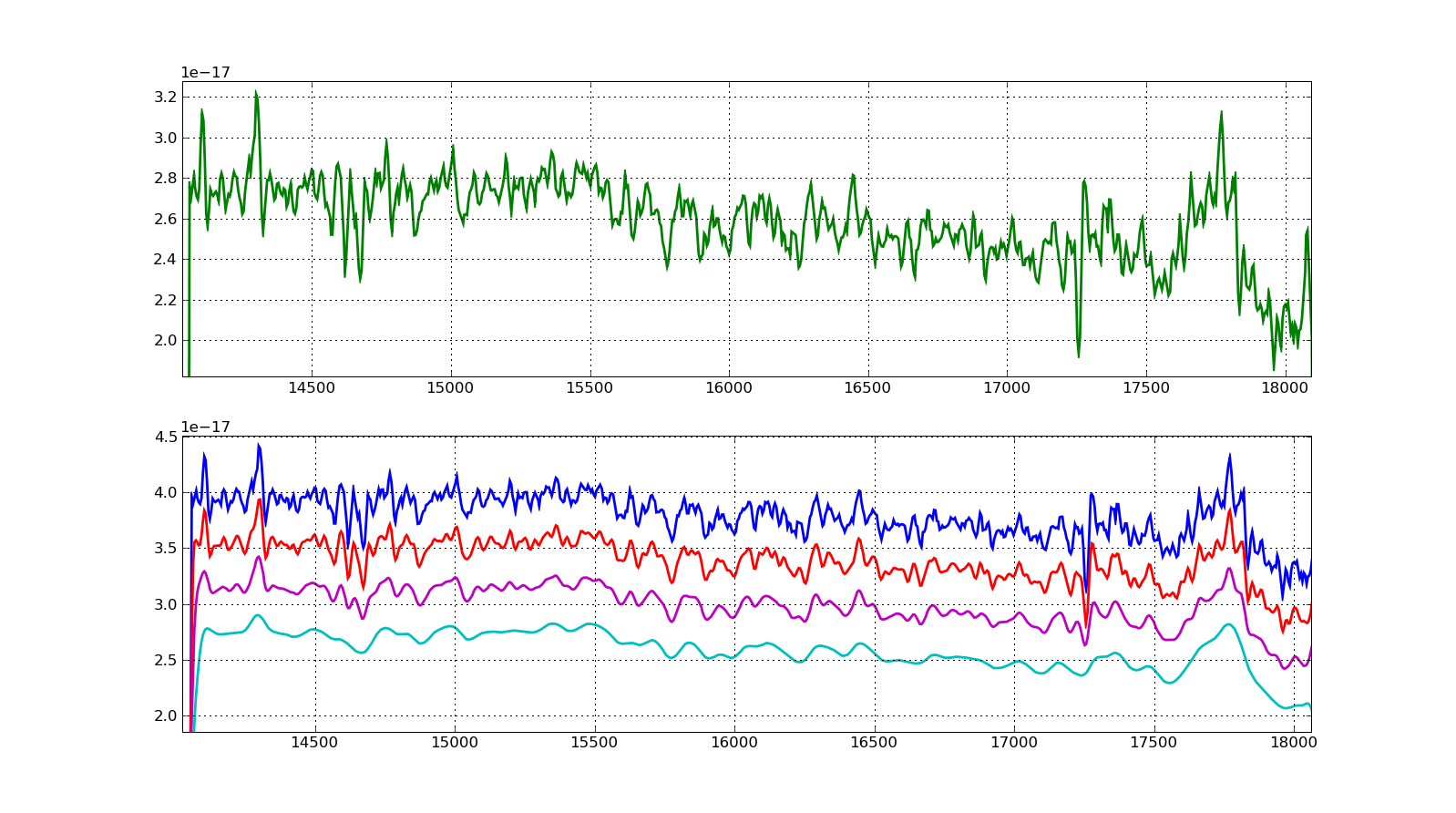Wavelets

Wavelet Transform of Astrophysical Data

The whole idea behind wavelets is the same as in the Fourier Transform: you measure how much your signal are correlated with some basis functions; the correlation-like coefficiens are the spectrogram. Fourier basis are good for harmonic oscilating signals that are infinite in time domain, something that is not so easy to achieve in practive. The wavelets are basis functions that are limited in the time domain, localized or with compact support as we say, i.e. they are non zero only in a small region in the domain. The transform are made with respect to two parameters in the wavelet, the scale and the shift:\psi is the mother wavelet and \psi_{a,b} are a shifted and scaled version of the mother wavelet; b is the shift, a the scale. So, in practice, you analyse your signal by continuing shifting and rescaling a function over the signal, something like sin/cos works for Fourier´s.

Well, the result is that your signal will be analysed locally for every shift (the wavelet is local) and at different scales. When the scale a is small, you are analysing your signal for small (fast, if domain is time) components, when a is large you are analysing for large (slow) components.

The discrete wavelet transform is when you take ´a´ to be discrete, usually a = 2**k where k is the wavelet order. In the plot below, the first component (rapid varying) is the differences between every 2**0=1 points, the second componet is the differences between 2**1=2 points, then 2**2=4, 8, 16.... So the signal is decomponsed in different scales. If you sum all the components you get the original signal exactly.The wavelets have zero mean, so if we take some out and reconstruct the signal we are not leaving out flux. The first component usually has almost only noise (we can measure that with a autocorrelation function) and we made noise reduction stuff just by selecting the wavelets coefficients we want; we are able to detect signals whithin the noise, but that's another history. Further, if you have a large (slow) component that you know what it is or that is boring you, you can leave it out.

I have written a Python module named Buwlets to do the wavelet transform of 1D and 2D data of any kind. I am particularly interested in applying it to astrophysical data but it can be applied to anything. If you are interested in the package please contact me; it is not avalilable in the site until I publish it, then it will be.

All the analysis done here were done with Buwlets. If you are interested, please, send me an email.The wavelet transform is a way of representing some signal based on orthogonal funcions (the wavelets) which may represent the information on the signal (they form a base). The wavelet can be used to extract information from the signal at different scales, either time, space or wavelength scales for example. It can be used, for example, to study galaxy morphology (click on the image). It is also very suitable for removing noise in contaminated data and for selecting specific features in spectra.

This example is a galaxy spectra which was decomposed with the wavelet transform and then reconstructed leaving out some wavelet coefficients. This can be done to separate different wavelength scales in the original signal. Also, depending on the noise type, they affect mostly some a determined scale and then can be taken out from the signal. The inverse wavelet transform is complete and unlike other filters, like mean, the signal features are preseved within scales.The transform applied to images separates differest spatial scales in the signal. In this example, the M51 galaxy, each wavelet coefficient shows different information, each one related to one spatial scale. In the first (upper left) only the smallest discernible scale in the signal is shown, while in the last (lower right) the overall spiral arms pattern are visible.Rio Grande, RS - Brasil 10 Dec 2023, 14h59m your IP: 44.212.94.18

updated Jun 2013, © Fabricio Ferrari
ferrari (at) ferrari pro br